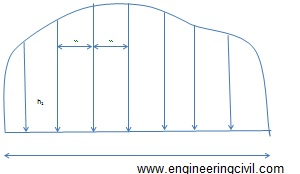Search

# Computation of Area from Plan

Posted in Soil Engineering, Student Corner |Email This Post |

Keywords: graphical methods, area computation, mid ordinate rule, trapezoid rule, Simpson’s rule, Parabolic rule

This article discusses the second method for computation of area (i.e., from previously plotted plan). You can read the first method ( computation of area using field notes) here.

Several methods can be used to determine the areas from plans. These methods are generally classified as graphical methods and instrumental methods

1) Graphical methods
These methods use the measured data from the existing plan. The areas may be calculated for the area as a whole or the areas is found by adding the areas of the skeleton and the area of the irregular strip between the skeleton and the boundary.

a) Entire areas
Generally used methods to calculate the area as whole is enumerated as follows:

i) By division of the area into triangles
In this method the total area is divided into a number of triangles. The base and altitude of each triangle are, measured and its area is found. When the boundaries are irregular, straight lines termed as ‘give and take’ or ‘equalizing lines’ replace them. These lines are so drawn that they exclude as much as they include as shown in figure.

ii) By division of the area into squares
This method is explains by figure. A piece of tracing paper is divided into squares of equal areas. This is place over the drawing. The total number of complete squares and fractions of squares formed along the boundaries are estimated in terms of full squares. The area of the plan is equal to the number of square’s multiplies by the area of one square.

iii) By division of the area into trapezoids
A number of equidistant parallel lines are drawn on a tracing paper and the tracing paper is placed over the plan so that the area is exactly enclosed between two parallel lines. Then the plotted area is divided into a number of strips. The curved ends of which are replaced by perpendicular ‘give and take’ lines. The sum of the base length of the rectangles thus obtains, multiplied by the common distance between the parallel lines gives the area of the plan.

b) Areas along boundaries
The areas enclosed between the adjacent survey lines and the curved boundaries are determined by using ordinated. The survey line is divided into a number of equal parts and the ordinate at each point is drawn and scaled. The area can be calculated by several methods as described below:

i) Mid ordinate rule
Ordinates are measured at the midpoint of each division.

Area= (h1+h2+h3+……+hn) L/n

Area= (h1+h2+h3+…..+hn) d

Where
h1,h2,h3,h4…..,hn= ordinates at the midpoint of each division
L= length of the base line
n= the number of equal parts into which the baselines is divided
d= the common distance between the ordinates=L/nFigure 1: Illustration of mid ordinate rule

ii) Trapezoid rule
This rule is more accurate than the mid-ordinate and the average ordinate rules. To apply this method, the whole area has to be divided into a number of trapezoids. The rule can be stated as ‘to the sum of the first and last ordinates, ass twice the sum of the intermediate ordinates. Then multiply the total sum with the common distance between the ordinates. The one half of this product gives the required area’

Area=d/2 {O0+On+2(O1+O2+….+On)}

When the baseline cuts the boundary at one or both ends, then O0 or On are zero. In that case O0 and On becomes zero in the equation above.

The trapezoid rule assumes that the boundaries between the ends of the ordinates are straight lines. The derivation of the formula is simple and can be tried out.

iii) Simpson’s rule or Parabolic rule
The rule may be stated as ‘to the sum of the first and last ordinates, add twice the sum of the remaining odd ordinates and four times the sum of all the even ordinates. Multiply the total sum thus obtained by one-third f the common distance between the ordinates. This gives the area requires’

Area-d/3 {O0+On+2 (O2+O4+O6+….) 4 (O1+O3+O5…)}

This method requires that there be an even number of divisions of the area. If there is odd number of divisions, the area of the last division must be calculated separately and added to the result obtained by applying the Simpson’s rule to the remaining even divisions.It is to be noted that an odd number of ordinates corresponds to an even number of divisions.

The second method to calculate area from a previously plotted plan (i.e. instrumental methods) is discussed here.

More Entries :
• Post a comment